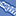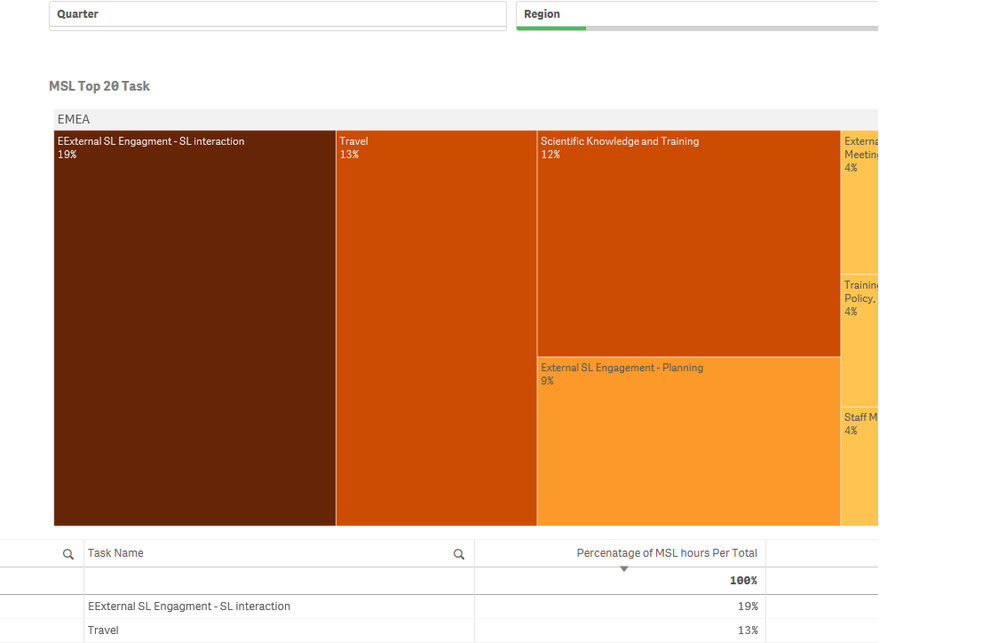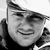# New to Qlik Sense

If you’re new to Qlik Sense, start with this Discussion Board and get up-to-speed quickly.

cancel
Showing results for
Did you mean:Creator II

## Calculating Percentage of Total By Regionin Qliksense

Hi,

I have to calculate percentage of MSL hours by total for each region, say in a Table or Treemap.

1. Dimension- Region,

2. Dimension -Task (Fixed to Top 20 ).

3. Measure is : =(sum({<FM={'MSL'}>}Q1hours)+sum({<FM={'MSL'}>}Q2hours)+sum({<FM={'MSL'}>}Q3hours)+sum({<FM={'MSL'}>}Q4hours)) /
(sum(total{<FM={'MSL'}>}Q1hours)+sum(total{<FM ={'MSL'}>}Q2hours)+sum(total{<FM ={'MSL'}>}Q3hours)+ sum(total{<FM ={'MSL'}>}Q4hours))

This gives me a decimal values and I convert it to Percentage.

I need to add Region also in code not sure how to do.

With above code I got below Screen ShotWhen I select Region in dashboard  The Region as EMEA , the shot comes like below and  that is what I want to be displayed.How should I display the data without selecting Region as filter in dashboard but rather as code in measure.

Regards,

Niha

1 Solution

Accepted SolutionsMVP

Try this measure

=(sum({<FM={'MSL'}>}Q1hours)+sum({<FM={'MSL'}>}Q2hours)+sum({<FM={'MSL'}>}Q3hours)+sum({<FM={'MSL'}>}Q4hours)) /
(sum(total <Region> {<FM={'MSL'}>}Q1hours)+sum(total <Region>{<FM ={'MSL'}>}Q2hours)+sum(total<Region>{<FM ={'MSL'}>}Q3hours)+ sum(total<Region>{<FM ={'MSL'}>}Q4hours))

2 RepliesMVP

Try this measure

=(sum({<FM={'MSL'}>}Q1hours)+sum({<FM={'MSL'}>}Q2hours)+sum({<FM={'MSL'}>}Q3hours)+sum({<FM={'MSL'}>}Q4hours)) /
(sum(total <Region> {<FM={'MSL'}>}Q1hours)+sum(total <Region>{<FM ={'MSL'}>}Q2hours)+sum(total<Region>{<FM ={'MSL'}>}Q3hours)+ sum(total<Region>{<FM ={'MSL'}>}Q4hours))Creator II
Author

Worked!Tags
Community Browser